# 30 Amazing Science Points with Force

Force

30 Amazing Science Points with Force(1) Force is an external effort in the form of push or pull which changes the state of rest or of uniform motion of a body along a straight line.

(2) Forces may be classified into two types…..(A) Contact Forces (B) Non – contact forces

(3) Contact force is that force which is exerted on an object by another when it is in direct contact with it.

Examples : Tension in a string, Frictional force between two surfaces etc.

(4) Non – contact force is force which is acting between two objects when they are not in contact.

Examples : Gravitational force of attraction between two bodies, electrical force of attraction/repulsion between charged objects, magnetic force of attraction or repulsion between two magnetized objects.

30 Amazing Science Points with Force

(5) General properties of non – contact forces

(a) are equal and opposite

(b) depend upon the distance between the objects

(c) depend upon the medium between the objects in case of electrical and magnetic forces except in case of gravitational force.

(6) Inertia is the property of a body to change its state of rest by itself.

(7) The SI unit of force is the newton (N).

(8) Definition of Newton : 1 N is that force which produces an acceleration of 1 metre per second square in a body of mass 1 kg.

In CGS system, the unit of force is dyne.

1 dyne is that force which produces an acceleration of 1 cm per second square in a body of mass 1 g.

1 N = 105 dyne

(9) The mass of a body is defined as the quantity of matter contained in the body. It is a scaler quantity. Its SI unit is kilogram (kg).

(10) The weight of a body on the earth is the gravitational pull exerted on it by the earth. Weight is a vector quantity. It is expressed as

w = mg

where m is the mass of the body and g is the acceleration due to gravity.

As g varies from place to place on the earth, the weight of a body of mass m also varies.

Its SI unit is the unit of force i.e. newton.

30 Amazing Science Points with Force(11) The gravitational units of force are kilogram force and gram force which are symbolically represented as kg f and g f respectively.

1 kg f = 9.8 N

1 g f = 980 dyne

TURNING FORCES

30 Amazing Science Points with Force

(12) A rigid body is one whose size and shape remains the same whatever force be applied. In actual practice, no material object is truly rigid.

(13) A rigid object has two types of motion.

(a) Translatory motion

(b) Rotatory motion

(14) In translatory motion, the object starts moving with all its particles along in the direction of force with the same speed.

(15) In rotatory motion, the object starts rotating about that point with its particles moving along concentric circles with different speeds.

30 Amazing Science Points with Force

(16) The turning effect of a force acting on an object about an axis is called moment of force or torque.

Mathematically, moment of force or the torque is the product of the applied force (F) and the perpendicular distance.

Torque = Force × Perpendicular distance

Moment of force or torque is a vector quantity. Its SI unit is newton – metre (N-m) and CGS unit is dyne – centimetre (dyne -cm).

1 N – m = 107dyne – cm(17) Moment of force is assigned a positive sign if the turning tendency of the force is anticlockwise and a negative sign if turning tendency of the force is clockwise.

(18) Couple is a pair of equal and opposite forces whose line of action of forces are not the same. Couple causes the object to execute rotational motion.

(19) Arm of the couple is the perpendicular distance between two equal and unlike parallel forces.

(20) The moment of a couple is equal to the product of either of the forces and the perpendicular distance.

Moment of a couple = either force × perpendicular distance

Moment of a couple = either force × couple arm

Moment of a couple = F × d

SI unit of moment of couple is newton metre denoted by N – m. Its CGS unit is dyne – cm.

30 Amazing Science Points with Force

(21) According to the principle of moments, the sum of anticlockwise moments of about any point must be equal to the sum of the clockwise moments of the forces about the same point.

(22) An object is said to be in equilibrium if a number of forces acting on it produce no change in its state of rest.

(23) An object is said to be in static equilibrium if it remains in the state of rest under the influence of applied forces.

(24) An object is said to be in dynamic equilibrium if it remains in the state of uniform motion.

(25) Under the action of a set of parallel forces, the two conditions for a body to be in equilibrium are :

(a) The resultant of all the forces acting on an object must be equal to zero.

(b) The resultant moment of all the forces acting on the object about a point should be zero.

30 Amazing Science Points with Force(26) Centre of gravity of an object is a point at which its total weight may be supposed to act.

(27) The position of the centre of gravity of some regular shaped objects having uniform density is given in the following table :

(a) Uniform rod – Mid point of rod

(b) Circular disc – Geometric centre

(c) Sphere (solid or hollow) – Geometric centre of the sphere

(d) Cylinder – Mid point on the axis of the cylinder

(e) Circular ring – Centre of the ring

(f) Rectangle or parallelogram – The point of intersection of the diagonals

(g) Triangular lamina – The point of intersection of medians

(h) Hollow cone – At a height h/3 from the base on its axis

(i) Solid cone – At a height h/4 from the base on its axis

(j) Cube or Cuboid – At the point of intersection of diagonals

(28) An object is said to be in stable equilibrium if it has a tendency to return to its original equilibrium position after being slightly disturbed.

Conditions for a body in stable equilibrium are :

(a) The centre of gravity of the object must be as low as possible.

(b) The vertical imaginary line from the centre of gravity must pass through the base of the object.

(29) An object is said to be in unstable equilibrium if it does not regain its original equilibrium position after being slightly disturbed.

Conditions for an object to be in unstable equilibrium are :

(a) The centre of gravity must be at its highest position.

(b) On tilting the object slightly, the centre of gravity is lowered.

(30) An object is said to be in neutral equilibrium, if after being slightly disturbed, the body does not have any tendency either to regain its original equilibrium position or to tilt further from its new position.

Conditions for an object to be in neutral equilibrium are :

(a) The centre of gravity should remain unaffected on tilting the body i.e. neither lowered nor raised.

(b) The vertical line joining the centre of gravity and centre of earth must always fall within the base of the object.30 Amazing Science Points with Force

UNIFORM CIRCULAR MOTION

(31) If an object moves on a circular path with a constant speed, its motion is said to be a uniform circular motion.

(32) In uniform circular motion, the speed of the body remains constant but direction changes continuously, due to which velocity changes continuously.

There is an acceleration in the uniform circular motion which is always directed towards the centre of the circle. It is called centripetal acceleration.

##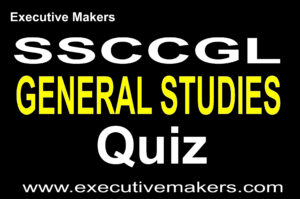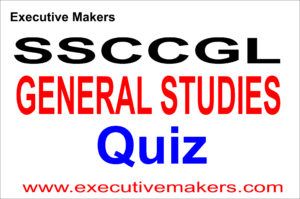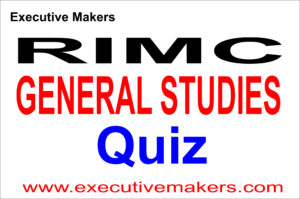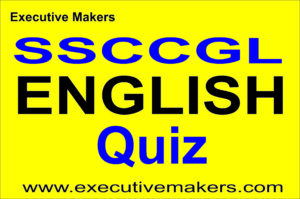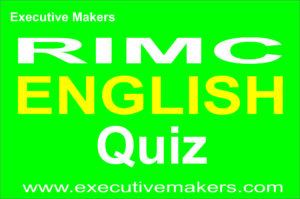## https://executivemakers.com/product/v96-up-police-set-papers-pdf## Mail at : executivemakerspvtltd12@gmail.com